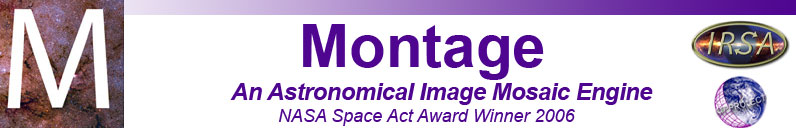# mCoverageCheck

Description:
mCoverageCheck can be used to subset an image metadata table (containing FITS/WCS information or image corners) by determining which records in the table represent images that overlap with a region definition (box or circle in the sky) given on the command line.
Syntax:
mCoverageCheck [-s statusfile] in.tbl out.tbl -mode <parameters>

Switches:

-s statusfile
Output and errors are sent to statusfile instead of to stdout
-mode
Can be one of:
• points (used to describe a convex polygon)
• box
• circle
• header (area is described in a header file)
• point (find images that overlap one particular point on the sky)
• cutout (operates like box mode, but returns metadata that represents what the input images would look like after being subset to exactly match the cutout area)

in.tbl
out.tbl
Output metadata table, to contain subset of in.tbl.
parameters
Depends on mode as following:
• points: ra1 dec1 ra2 dec2 ... raN decN (must be at least 3 vertices)
• box: ra dec xsize [ysize [rotation]]
where ra, dec is the center of the box, and size is in degrees.
• circle: ra dec radius
where ra, dec is the center of the circle, and radius is in degrees (default is 0, in which case it's treated like a point search).
• point: ra dec
• header: region.hdr (path to an ASCII header template that defines the region of interest)
• cutout: ra dec xsize [ysize]
where ra, dec is the center of the cutout area, and size is in degrees.
Results:
The output file out.tbl is created, with nimages records.

Examples:

As input, we use this table of 2MASS atlas images. The images in this table are in two "clumps" on the sky: 8 overlapping images near M51 and separated by several degrees from the other 6 overlapping images near M100. Here we will show several ways of isolating the first set.

\$ mCoverageCheck images.tbl circle.tbl -circle 202.48417 47.23056 1.0
[struct stat="OK", count="8"]
\$ mCoverageCheck images.tbl box.tbl -box 202.48417 47.23056 1.0 1.0
[struct stat="OK", count="8"]
\$ mCoverageCheck images.tbl points.tbl -points 203.213572 46.728255 201.754768 46.728255 201.740876 47.728147 203.227464 47.728147
[struct stat="OK", count="8"]
\$ mCoverageCheck images.tbl point.tbl -point 262.78208 -35.03049
[struct stat="OK", count="1"]

The first three methods results in this output table containing only 8 records out of the original 14 (the last is just the one image at the center).

Return Codes:

• [struct stat="OK", count=n]
• [struct stat="ERROR", msg="Usage: mCoverageCheck [-s statusfile] in.tbl out.tbl -<mode> <parameters> [where mode can be 'points', 'box', 'circle', 'header', 'point' or 'cutout'"]
• [struct stat="ERROR", msg="Cannot open status file: statusfile"]
• [struct stat="ERROR", msg="Usage: Input table file (filename) does not exist"]
• [struct stat="ERROR", msg="Invalid region definition mode: string"]
• [struct stat="ERROR", msg="Too few vertices for region (must be at least three)"]
• [struct stat="ERROR", msg="Longitude n (string) cannot be interpreted as a real number"]
• [struct stat="ERROR", msg="Latitude n (string) cannot be interpreted as a real number"]
• [struct stat="ERROR", msg="Failed to find bounding polygon for points"]
• [struct stat="ERROR", msg="Too few arguments for box or cutout (must at least have center and size)"]
• [struct stat="ERROR", msg="Center RA string (string) cannot be interpreted as a real number"]
• [struct stat="ERROR", msg="Center Dec string (string) cannot be interpreted as a real number"]
• [struct stat="ERROR", msg="X box size string (string) cannot be interpreted as a real number"]
• [struct stat="ERROR", msg="X box size (string) must be a positive number"]
• [struct stat="ERROR", msg="Y box size string (string) cannot be interpreted as a real number"]
• [struct stat="ERROR", msg="Y box size (string) must be a positive number"]
• [struct stat="ERROR", msg="Box rotation string (string) cannot be interpreted as a real number"]
• [struct stat="ERROR", msg="Must give header file name"]
• [struct stat="ERROR", msg="Failed to find bounding polygon for points"]
• [struct stat="ERROR", msg="Too few arguments for circle (must at least have center)"]
• [struct stat="ERROR", msg="Circle radius string (string) cannot be interpreted as a real number"]
• [struct stat="ERROR", msg="Too few arguments for point (must have coordinates)"]
• [struct stat="ERROR", msg="Point RA string (string) cannot be interpreted as a real number"]
• [struct stat="ERROR", msg="Point Dec string (string) cannot be interpreted as a real number"]
• [struct stat="ERROR", msg="Error opening table filename"]
• [struct stat="ERROR", msg="Cannot create output file filename"]
• [struct stat="ERROR", msg="Need either WCS or corner columns."]
• [struct stat="ERROR", msg="Failed to create wcs structure for record n"]